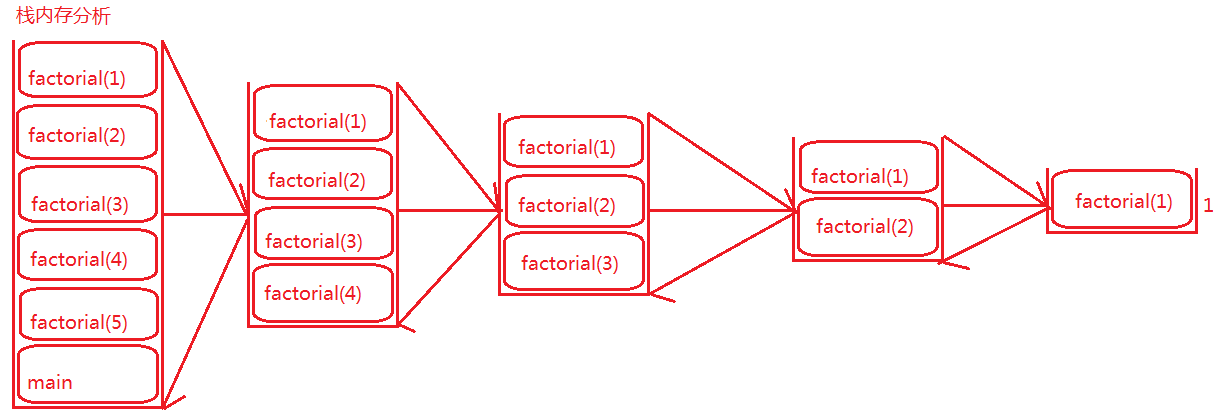Java中递归和循环的优劣Java中递归和循环的优劣

1、递归头：即什么时候不调用自身方法，也就是递归的结束条件。如果没有递归头，程序将陷入死循环。
2、递归体：即什么时候需要调用自身方法。

 1 2 3 4 5 6 7 8 9 10 11 12 13 14 15 16 17 18 19 20 21 22 23 24 25 26 27 28 29 30 31 32 33 34 35 36 37 38 39 40 `package` `com.bjwyj.method;` `/**` ` ``* 递归和循环的比较` ` ``* @author 吴永吉` ` ``*` ` ``*/` `public` `class` `TestRecursion {` `    ``public` `static` `void` `main(String[] args) {` `        ``//以下调用System下的currentTimeMillis()方法只是为了说明递归调用比循环调用更耗时` `        ``long` `l1 = System.currentTimeMillis();  ` `        ``System.out.println(factorial(``5``));` `        ``long` `l2 = System.currentTimeMillis();` `        ``System.out.println(``"递归计算阶乘耗时："``+(l2-l1));` `        ` `        ``System.out.println(``"\$\$\$\$\$\$\$\$\$\$\$\$\$\$\$\$\$\$\$\$\$\$\$\$\$\$\$\$\$\$\$\$\$\$"``);` `        ``long` `time1 = System.currentTimeMillis();` `        ``System.out.println(factorialLoop(``5``));` `        ``long` `time2 = System.currentTimeMillis();` `        ``System.out.println(``"循环计算阶乘耗时："``+(time2-time1));` `    ``}` `    ` `    ``//使用递归定义计算阶乘的方法` `    ``public` `static` `long` `factorial(``int` `num) {` `        ``if``(num==``1``) {    ``//递归头` `            ``return` `1``;` `        ``}``else` `{` `            ``return` `num*factorial(num-``1``);    ``//递归体` `        ``}` `    ``}` `    ` `    ``//使用循环定义计算阶乘的方法` `    ``public` `static` `long` `factorialLoop(``int` `n) {` `        ``int` `result = ``1``; ``//接收计算结果` `        ``while``(n>``1``) {` `            ``result *= n*(n-``1``);  ``//实现计算结果的累乘操作` `            ``n -= ``2``; ``//每次减去2，实现数字的迭代操作` `        ``}` `        ``return` `result;` `    ``}` `}`

 1 2 3 4 5 `120` `递归计算阶乘耗时：``1` `\$\$\$\$\$\$\$\$\$\$\$\$\$\$\$\$\$\$\$\$\$\$\$\$\$\$\$\$\$\$\$\$\$\$` `120` `循环计算阶乘耗时：``0`